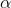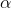# Angular Speed of Rollers

annao

## Homework Statement

A plank of wood sits on top of two rollers with radius of 44.4 cm. The plank is pulled forward so it moves at a constant acceleration of 2.24 m/s^2, starting from rest, and the rollers roll without slipping along the ground and in contact with the plank. What is the angular speed of the rollers after 1.14 s? Express your answer in rad/s.

## Homework Equations=a/r
w=wo+t

## The Attempt at a Solution

wo=0 rad/s; t=1.14 s; a=2.24 m/s^2; r=0.444 m=a/r = 2.24/0.444 = 5.045 rad/s^2

w=wo+t = 0+5.045*1.14

Am I on the right track? Do I need to divide by 2 because there are two rollers?

Thank you

## Answers and Replies

Cutter Ketch
You are definitely on the right track. In fact you’ve done it almost comletely right.

You do not need to divide by 2 because there are two rollers. They say two rollers just because it is more natural than saying the plank is balancing on one roller.

However you have missed a factor of 2 for an unrelated and kind of tricky reason. Free rollers don’t stay put relative to the ground, but they also don’t travel with the plank. When you say α = a/r, what exactly is a?

Consider 2 examples If the rollers were rigidly glued to the bottom of the plank and the ground was made slippery, when the plank accelerated with linear acceleration = a the top of the roller would have the full linear acceleration of the plank = a, but would have no angular acceleration. So α ≠ a/r in this case a/r. Similarly, if the rollers turned on an axles rigidly attached to the ground, the contact point would accelerate with the plank. This time, however, α = a/r.

What happens with free rollers?

annao
I think I have it figured out, the answer is divided by two because the top rolls have twice the speed as the centre.